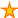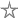# Calculate a persons age given the DOB

13 votes:20,851 views      No comments
by Allen Browne, 20 April 2005    (for Access 95+)

### Microsoft Access: VBA Programming Code

Provided by Allen Browne, allenbrowne.com

# Age() Function

Given a person's date-of-birth, how do you calculate their age? These examples do not work reliably:

```    Format(Date() - DOB, "yyyy")
DateDiff("y", DOB, Date)
Int(DateDiff("d", DOB, Date)/365.25)```

DateDiff("y", ..., ...) merely subtracts the year parts of the dates, without reference to the month or day. This means we need to subtract one if the person has not has their birthday this year. The following expression returns True if the person has not had their birthday this year:

`    DateSerial(Year(Date), Month(DOB), Day(DOB)) > Date`

True equates to -1, so by adding this expression, Access subtracts one if the birthday hasn't occurred.

The function is therefore:

```Function Age(varDOB As Variant, Optional varAsOf As Variant) As Variant
'Purpose:   Return the Age in years.
'Arguments: varDOB = Date Of Birth
'           varAsOf = the date to calculate the age at, or today if missing.
'Return:    Whole number of years.
Dim dtDOB As Date
Dim dtAsOf As Date
Dim dtBDay As Date  'Birthday in the year of calculation.

Age = Null          'Initialize to Null

'Validate parameters
If IsDate(varDOB) Then
dtDOB = varDOB

If Not IsDate(varAsOf) Then  'Date to calculate age from.
dtAsOf = Date
Else
dtAsOf = varAsOf
End If

If dtAsOf >= dtDOB Then      'Calculate only if it's after person was born.
dtBDay = DateSerial(Year(dtAsOf), Month(dtDOB), Day(dtDOB))
Age = DateDiff("yyyy", dtDOB, dtAsOf) + (dtBDay > dtAsOf)
End If
End If
End Function```

 Home Index of tips Top

Rate this article:This is a cached tutorial, reproduced with permission.